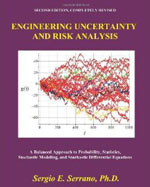ENGINEERING UNCERTAINTY AND RISK ANALYSIS, Second Edition. A Balanced Approach to Probability, Statistics, Stochastic Modeling, and Stochastic Differential Equations. - Maplesoft Books - Maple Books, Maple Resources and Math BooksHome : Maplesoft Books : ENGINEERING UNCERTAINTY AND RISK ANALYSIS, Second Edition. A Balanced Approach to Probability, Statistics, Stochastic Modeling, and Stochastic Differential Equations.Details Search# ENGINEERING UNCERTAINTY AND RISK ANALYSIS, Second Edition. A Balanced Approach to Probability, Statistics, Stochastic Modeling, and Stochastic Differential Equations.

## 2011